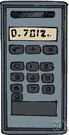# calculator

(redirected from Graphics calculator)
Also found in: Thesaurus, Encyclopedia.

## cal·cu·la·tor

(kăl′kyə-lā′tər)
n.
1. One that calculates, as:
a. An electronic or mechanical device for the performance of mathematical computations.
b. A person who operates such a machine or otherwise makes calculations.
2. A set of mathematical tables used to aid in calculating.

## calculator

(ˈkælkjʊˌleɪtə)
n
1. (Mathematics) a device for performing mathematical calculations, esp an electronic device that can be held in the hand
2. a person or thing that calculates
3. (Mathematics) a set of tables used as an aid to calculations

## cal•cu•la•tor

(ˈkæl kyəˌleɪ tər)

n.
1. a small, hand-operated electronic or mechanical device that performs calculations.
2. a set of tables that facilitate calculation.
3. a person who calculates.
[1375–1425]
ThesaurusAntonymsRelated WordsSynonymsLegend:
 Noun 1calculator - an expert at calculation (or at operating calculating machines)expert - a person with special knowledge or ability who performs skillfullyadder - a person who adds numbersnumber cruncher - someone able to perform complex and lengthy calculationsactuary, statistician - someone versed in the collection and interpretation of numerical data (especially someone who uses statistics to calculate insurance premiums)subtracter - a person who subtracts numbers 2calculator - a small machine that is used for mathematical calculationscalculating machineabacus - a calculator that performs arithmetic functions by manually sliding counters on rods or in groovesadder - a machine that adds numbersadding machine, totaliser, totalizer - a calculator that performs simple arithmetic functionstabulator, counter - a calculator that keeps a record of the number of times something happenshand calculator, pocket calculator - a calculator small enough to hold in the hand or carry in a pocketmachine - any mechanical or electrical device that transmits or modifies energy to perform or assist in the performance of human tasksNapier's bones, Napier's rods - a set of graduated rods formerly used to do multiplication and division by a method invented by John Napierquipu - calculator consisting of a cord with attached cords; used by ancient Peruvians for calculating and keeping recordssubtracter - a machine that subtracts numbers
Translations
آلَةٌ حَاسِبَةحاسِب، حاسِبَه، عَدّاد
kalkulačkapočítačka
kalkulator
számológépzsebszámológép
reiknivél, tölva

계산기
calculator
kalkulačka
kalkulator
računalo
miniräknareräknarekalkylator
เครื่องคิดเลข
hesap makinesihesap makinası
máy tính

## calculator

[ˈkælkjʊleɪtəʳ] N (= machine) →

## calculator

[ˈkælkjʊleɪtər] n
(also pocket calculator) →
(on computer)
(= formula) →

## calculator

n
(= machine)Rechner m
(= person)Kalkulator(in) m(f), → Rechnungsbeamte(r) m/-beamtin f
(= table of figures)Rechentabelle f

## calculator

[ˈkælkjʊˌleɪtəʳ] n

## calculate

(ˈkӕlkjuleit) verb
to count or estimate, using numbers. Calculate the number of days in a century.
ˌcalcuˈlation noun
ˈcalculator noun
a machine for calculating. Use a calculator for adding all those numbers.

## calculator

kalkulačka lommeregner laskin kalkulator 計算機 계산기 miniräknare เครื่องคิดเลข máy tính
References in periodicals archive ?
On a graphics calculator, identities can be powerfully explored by comparing tables and graphs for functions thought to be the same.
Among the topics are the influence of professional learning on secondary science teachers' ways of improving their subject teaching in Bangladesh, attitudes toward and engagement with the graphics calculator among senior secondary mathematics students in Singapore, a socio-historical interpretation of current curriculum and pedagogy reform in Vietnamese early childhood education, a railway enterprise-run school as a case study of the new governance in Chinese public education reform, and students' educational experiences in a weekend Chinese school in Victoria (Australia).
We need students who can reason, who are creative and who have a logical understanding of their subjects." One of the devices presented by Seth was a graphics calculator, Casio 9860 Plus, which has been designed for mathematical modelling activities in the classroom.
For comparison, items which required the student to read data from a graph were considered to be of Type 10 ("Task requires that a representation of a graphics calculator screen will be interpreted").
She wants to study Alevel maths and has been told she needs to have a graphics calculator.
Impact is defined to mean that "a CAS-user would have access to a more efficient solution strategy than a graphics calculator user ...
Calculator use is assumed: the graphics calculator for Mathematical Methods, and the CAS calculator for Mathematical Methods CAS.
Physical chemistry is used to explain the principles of quantified energy level transitions in AAS while mathematical modeling and graphics calculator manipulation is used to analyze AAS data.
The effect of graphics calculator use on precalculus students' understanding of polynomial, rational, and exponential functions.
In this paper, we show that an effective use of a graphics calculator in an introductory statistics course can enhance students' performance in the course.
Concordia's David Hambly ('Studies of Acid Diffusion in Thin Polymer Films') took the first prize, a Hewlett-Packard graphics calculator worth more than \$500.
In addition, the extensive use of the graphics calculator as a tool for learning and doing mathematics helps students whose limited computational abilities previously prevented them from advancing in the study of important mathematics.

Site: Follow: Share:
Open / Close